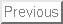Mathematics 15.3 - Term Syllabus

The Undergraduate Program Committee

Date: May 12, 1999

Lecture Topics/Sections Some Standard Examples/Concepts
Day 1   Overview of expectations for course, revised syllabus and discussion. Circuit problem.
Day 2   Electric circuits. Series solution to first order ODEs.
Day 3   Taylor Series, geometric series.
Day 4   Discussion of homework. Absolute vs conditional convergence. Integral tests.
Day 5   Ratio test, comparison test.
Day 6   The mathematician's view of simple closed circuits. Reality versus its model. (General second order ODE with constant coefficients. Homogeneous and inhomogeneous. Main theorem of ODE's.)
Day 7   Matrix version of the circuit problem. Comparison with diagonal matrices. Exponential of matrix.
Day 8   Matrix algebra, properties of a matrix not dependent on coordinates, computation of eigenvalues. Solve Wednesday's circuit problem.
Day 9   Relax and review.
Day 10   The heat equation on a wire. Setting it up.
Day 11   Turning the heat equation into an eigenvalue problem. Possible solutions.
Day 12   Student presentation of models. Three ways to look at a chime.
Day 13   General discussion of Fourier series. Idea of vector spaces of functions, linear independence, completeness, etc.
Day 14   Discussion of colloquium with Beth Bradley. [on an application of the wave equation.]
Day 15   Bring your solutions to the wave equation for your chime. Student presentations of solutions. Oscilloscope will do Fourier analysis and tell you what frequencies are present.
Day 16   Guest demo: Building guitars using the wave equation. The round drumhead. Mook's vibrating membrane.
Day 17   Guest presentation: analysis of oboe sounds and questions for speaker. In class modelling problem: human voice.
Day 18   Relax and review.
Day 19   Set up of Schrodinger's equation. Probability distributions. Discussion of symmetry issues for that and the spring model. Idea of symmetry group.
Day 20   Separation of variables. Eigenvalue problem associated to hydrogen.
Day 21   The group of rotations, invariant subspaces, representations.
Day 22   Student presentation of homework problems
Day 23   Schur's lemma, intro to spherical polynomials
Day 24   the spherical polynomials, intro to last assignment
Day 25   Homework: compute the allowable eigenvalues for hydrogen. Can you adjust parameters so that they match the real ones?
Day 26   Demo: look at Balmer lines. Discussion of final exam.
Day 27   Surveys,-pass out take-home; collect homework from Schrodinger's equation (all).
Day 28